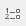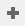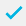# Date function examples

## Using a date function to determine the day of the week of a date

This example shows the use of the date function weekday. You define a Derived Single Response variable to work out the day of the week for a particular date.

1. Clickto display the Variables window.
2. Clickto add a new variable.
3. Specify the Variable Details:
• Name: V6
• Label: Day of the week restaurant visited
• Type: Derived (the variable will derive its data from other existing variables).
• Response: Single (each respondent will fall into only one of the new codes, as there is only one date of visit per case).
4. Specify the Code Details:
1. Clickto save the variable. The variable can then be used in tables and charts in the usual way.

## Calculating age from a date of birth

These instructions tell you how to create variables to calculate the respondent’s age from their date of birth and then put the respondents into age bands, based on the difference between a date of birth variable and the date function today. The instructions also apply if you have another date question in the questionnaire, which you want to use instead of today.

For this example, the date of birth variable is Q1a. It assumes a four-digit year.

1. Clickto display the Variables window.
2. Clickto add a new variable.
3. Specify the Variable Details as:
• Name: Age
• Label: Calculated age in years
• Type: Derived (the variable will derive its data from other existing variables).
• Response: Quantity (the response will be a numeric value for each case).
1. Specify the Code Values to calculate the number of years. Note the use of \ to extract the whole number of years.
1. Clickto save the variable. You can then use this to analyse respondents’ ages.
2. The Not Asked value is specified to ensure that the calculation is only performed if data exists for the date question.
3. To band the respondents into age groups, create a new variable:
• Name: Agegroup
• Label: Age groups
• Type: Derived
• Response: Single (each respondent will only fall into one of the new codes).
4. Specify the Code Details:
1. The codes are assigned in order, so all responses that have not been sorted into previous bands appear as 65 plus.
2. Clickto save the variable.
Contents